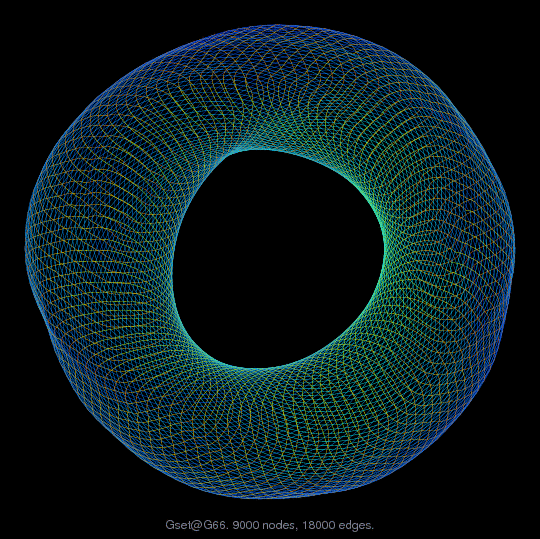Matrix: Gset/G66

Description: 2D torus, 100-by-90(undirected graph drawing)• Matrix group: Gset
• download as a MATLAB mat-file, file size: 70 KB. Use UFget(531) or UFget('Gset/G66') in MATLAB.

 Matrix properties number of rows 9,000 number of columns 9,000 nonzeros 36,000 # strongly connected comp. 1 explicit zero entries 0 nonzero pattern symmetry symmetric numeric value symmetry symmetric type integer structure symmetric Cholesky candidate? no positive definite? no

 author C. Helmberg editor Y. Ye date 1996 kind undirected weighted random graph 2D/3D problem? no

 SVD-based statistics: norm(A) 3.58207 min(svd(A)) 0.000214411 cond(A) 16706.5 rank(A) 9,000 null space dimension 0 full numerical rank? yes

 singular values (MAT file): click here SVD method used: s = svd (full (A)) ; status: ok